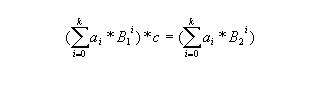# 2296. Base equality

Numerous are the moments I as a programmer have been frustrated by the tedious conversions between decimal numbers and hexadecimal ones. Why have we chosen 10 as a base in our everyday numerical presentations, when 16 seems so practically appealing? Obviously because everyone is not the computer geek I am. Maybe some day the world will fully realise the benefits of the hexadecimal system. In the meantime I have to learn to master the base conversions since most of the time numbers do not resemble one another in different bases.

Sometimes peculiar relationships emerge among the different base representations of numbers though. For instance, I noticed just the other day that 1040(10) * 4 = 1040(16), i.e. (110^3+010^2+410^1+010^0)4=(116^3+016^2+416^1+0*16^0). It made me wonder how often this is the case, that is, the digits of a number in one base, are exactly the same as the digits of a multiple of the number in another base. Formally, let B1 < B2 be positive integers, and a0,a1,…,ak be integers in [0…B1-1]. For which ai’s is there a positive integer c such that### 输入格式

On the first line of input is a positive integer n telling the number of test cases that follow. Each test case is on a line of its own and consists of two integer bases B1, B2, 9 <= B1 < B2 <= 100, and two integer range elements, r1, and r2, 0 < r1 < r2 <= 10000. Notice that all numbers in the input are given in the base 10.

### 输出格式

For each test case, there should be one row containing the largest integer i, fulfilling r1 < i < r2, for which there is a positive integer c such that the digits of i in the base B1, are exactly the same as the digits of i*c in the base B2. If no such integer i exists, output the text ‘Non-existent.’.

### 样例

Input
4
10 16 1 2000
10 16 1 4999
10 14 10 9999
11 14 10 9999

Output
1040
4240
Non-existent.
9240


14 人解决，20 人已尝试。

14 份提交通过，共有 25 份提交。

4.9 EMB 奖励。